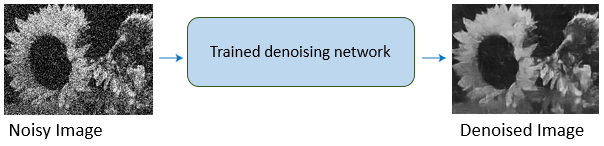# Deep Learning for Image Processing

Perform image processing tasks, such as removing image noise and creating high-resolution images from low-resolutions images, using convolutional neural networks (requires Deep Learning Toolbox™)

Deep learning uses neural networks to learn useful representations of features directly from data. For example, you can use a pretrained neural network to identify and remove artifacts like noise from images.## Functions

expand all

 `augmentedImageDatastore` Transform batches to augment image data `bigimageDatastore` Datastore to manage blocks of big image data `denoisingImageDatastore` Denoising image datastore `imageDatastore` Datastore for image data `randomPatchExtractionDatastore` Datastore for extracting random 2-D or 3-D random patches from images or pixel label images `transform` Transform datastore `combine` Combine data from multiple datastores
 `jitterColorHSV` Randomly alter color of pixels `centerCropWindow2d` Create rectangular center cropping window `centerCropWindow3d` Create cuboidal center cropping window `randomCropWindow2d` Create randomized rectangular cropping window `randomCropWindow3d` Create randomized cuboidal cropping window `Rectangle` Spatial extents of 2-D rectangular region `Cuboid` Spatial extents of 3-D cuboidal region `randomAffine2d` Create randomized 2-D affine transformation `randomAffine3d` Create randomized 3-D affine transformation `affineOutputView` Create output view for warping images
 `denoiseImage` Denoise image using deep neural network `denoisingNetwork` Get image denoising network `dnCNNLayers` Get denoising convolutional neural network layers

## Topics

### Preprocess Images for Deep Learning

Datastores for Deep Learning (Deep Learning Toolbox)

Learn how to use datastores in deep learning applications.

Augment Images for Deep Learning Workflows Using Image Processing Toolbox (Deep Learning Toolbox)

This example shows how MATLAB® and Image Processing Toolbox™ can perform common kinds of image augmentation as part of deep learning workflows.

Preprocess Images for Deep Learning (Deep Learning Toolbox)

Learn how to resize images for training, prediction, and classification, and how to preprocess images using data augmentation, transformations, and specialized datastores.

Preprocess Volumes for Deep Learning (Deep Learning Toolbox)

Read and preprocess volumetric image and label data for 3-D deep learning.

### Denoise Images Using Deep Learning

Train and Apply Denoising Neural Networks

Use a pretrained neural network to remove Gaussian noise from a grayscale image, or train your own network using predefined layers.

Remove Noise from Color Image Using Pretrained Neural Network

This example shows how to remove Gaussian noise from an RGB image by using a pretrained denoising neural network on each color channel independently.

Prepare Datastore for Image-to-Image Regression (Deep Learning Toolbox)

This example shows how to prepare a datastore for training an image-to-image regression network using the `transform` and `combine` functions of `ImageDatastore`.

### Deep Learning in MATLAB

Deep Learning in MATLAB (Deep Learning Toolbox)

Discover deep learning capabilities in MATLAB® using convolutional neural networks for classification and regression, including pretrained networks and transfer learning, and training on GPUs, CPUs, clusters, and clouds.

Pretrained Deep Neural Networks (Deep Learning Toolbox)

Learn how to download and use pretrained convolutional neural networks for classification, transfer learning and feature extraction.

Semantic Segmentation Using Deep Learning (Computer Vision Toolbox)

This example shows how to train a semantic segmentation network using deep learning.